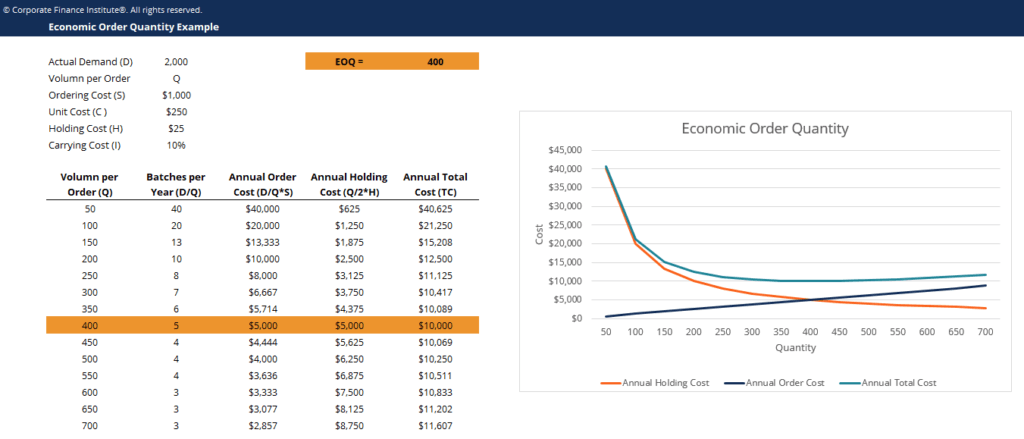# Economic Order Quantity Template

## Economic Order Quantity Template

This economic order quantity template helps you identify the optimal order quantity which minimizes the cost of ordering and holding inventory.

This is what the economic order quantity template looks like:### Download the Free Template

Enter your name and email in the form below and download the free template now!

### Economic Order Quantity Template

Download the free Excel template now to advance your finance knowledge!

Economic Order Quantity (EOQ) is a measurement used in the field of Operations, Logistics, and Supply Management. In essence, EOQ is a tool used to determine the volume and frequency of orders required to satisfy a given level of demand while minimizing the cost per order.

The Economic Order Quantity is a set point designed to help companies minimize the cost of ordering and holding inventory. The cost of ordering an inventory falls with the increase in ordering volume due to purchasing on economies of scale. However, as the size of inventory grows, the cost of holding the inventory rises. EOQ is the exact point that minimizes both these inversely related costs.

### EOQ Formula

The Economic Order Quantity formula is calculated by minimizing the total cost per order by setting the first order derivative to zero. The components of the formula that make up the total cost per order are the cost of holding inventory and the cost of ordering that inventory. The key notations in understanding the EOQ formula are as follows:

Components of the EOQ Formula:

D: Annual Quantity Demanded

Q: Volume per Order

S: Ordering Cost (Fixed Cost)

C: Unit Cost (Variable Cost)

H: Holding Cost (Variable Cost)

i: Carrying Cost (Interest Rate)

### More Free Templates

For more resources, check out our business templates library to download numerous free Excel modeling, PowerPoint presentation and Word document templates.

• Excel Modeling Templates
• PowerPoint Presentation Templates
• Transaction Document Templates

### Financial Analyst Certification

Become a certified Financial Modeling and Valuation Analyst (FMVA)® by completing CFI’s online financial modeling classes and training program!